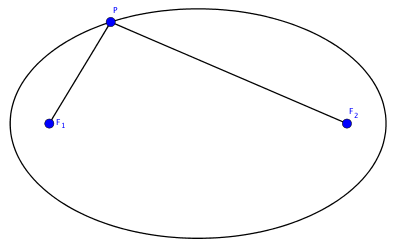# Lambda Calculus

This post is my note for What is seminar on Lambda Calculus.

Lambda calculus was created by Alonzo Church in the 1930s, and was used by him to solve Entscheidungsproblem in 1936, which is related to Hilbert's tenth problem. In the same year, Alan Turing independently solved Entscheidungsproblem using his invention Turing machine. Shortly after, Turing realized that these two models are actually equivalent as models of computation.

In this note, I will first give the formal definition of lambda expressions. Then with the help of Python, I am going to show how to do Boolean algebra and basic arithmetic using lambda calculus, which to some extend gives an illustration that Turing machine and lambda calculus are equivalent.

## Definition

Lambda calculus consists of lambda expressions and operations on them. There are three basic elements in Lambda expression:

1. variables: x, y, z, ...
2. symbols in abstraction: λ and .
3. parentheses for association: ()

# Cassini Ovals

This post is my note for What is seminar on Cassini Ovals.

### Definition

An ellipse is a geometric object formed by locus of points which have fixed sum of distances to two fixes foci.In the above figure, we can express the definition of ellipses in one simple equation:

$$|PF_1|+|PF_2|=c,$$

for some $c>0$. What if we change the addition in the above equation to multiplication? This comes the definition of Cassini ovals.

# Leetcode Contest 38

1. 第一个数 X 第二个数 X 第三个数
2. 倒数第一个数 X 倒数第二个数 X 倒数第三个数
3. 第一个数 X 倒数第一个数 X 倒数第二个数
4. 第一个数 X 第二个数 X 倒数第一个数
class Solution(object):
def maximumProduct(self, nums):
"""
:type nums: List[int]
:rtype: int
"""
nums.sort()
return max(nums*nums*nums,
nums[-1]*nums[-2]*nums[-3],
nums*nums[-1]*nums[-2],
nums*nums*nums[-1])


This post is my note for What is…? seminar on Rademacher function.

For a real number $x$ in $[0, 1]$, let $(a_1(x), a_2(x), \cdots, a_n(x), \cdots)$ be its binary representation. That is to say, $\begin{equation} x = \sum_{n=1}^\infty \frac{a_n(x)}{2^n}. \label{20170623-1} \end{equation}$

For some $x$, there might be two possible binary representation. Take $\frac{1}{2}$ for example, it can be represented as $(1, 0, 0, \cdots)$ or $(0, 1, 1, \cdots)$. In this situation, we always prefer the former representation, in which all terms become $0$ eventually.

Definition 1. Let $n \ge 1$ be an integer. The $n$-th Rademacher function $r_n: [0, 1] \to \{-1,1\}$ is defined to be $r_n(x) = 1 - 2a_n(x).$

# Lüroth expansion

This post is my note for What is…? seminar on Lüroth expansion.

Definition 1. A Lüroth expansion of a real number $x \in (0, 1]$ is a (possibly) infinite sequence $(a_1, a_2, \cdots, a_n, \cdots)$ with $a_n \in \N$ and $a_n \ge 2$ for all $n \ge 1$ such that $\begin{equation} x = \frac{1}{a_1} + \frac{1}{a_1(a_1-1)a_2} + \cdots + \frac{1}{a_1(a_1-1)\cdots a_{n-1}(a_{n-1}-1)a_n} + \cdots \end{equation}$

By abuse of notation, we will something write the right hand side of Definition 1 as $(a_1, a_2, \cdots, a_n, \cdots)$.

Given a system of representation of real numbers in $(0, 1]$, we need to determine whether these representations are 1 to 1 corresponding the numbers in $(0, 1]$. Aslo we should study the (Lüroth) shift map: $\begin{equation} T: (a_1, a_2, \cdots, a_n, \cdots) \mapsto (a_2, \cdots, a_n, \cdots). \label{20170620-2} \end{equation}$

# Leetcode Contest 37

class Solution(object):
def maxDistance(self, arrays):
"""
:type arrays: List[List[int]]
:rtype: int
"""
m = []
for i, a in enumerate(arrays):
m.append((a, i))
m.append((a[-1], i))
m.sort(key=lambda x: x)
if m!=m[-1]:
return m[-1]-m
return max(m[-2]-m, m[-1]-m)


# Leetcode Contest 35

class Solution(object):
def canPlaceFlowers(self, flowerbed, n):
"""
:type flowerbed: List[int]
:type n: int
:rtype: bool
"""
cnt = 0
for i in xrange(len(flowerbed)):
if flowerbed[i] == 1:
continue
if i-1>=0 and flowerbed[i-1]==1:
continue
if i+1<len(flowerbed) and flowerbed[i+1]==1:
continue
flowerbed[i] = 1
cnt += 1
return cnt>=n


# Leetcode Contest 33

class Solution(object):
def findLHS(self, nums):
"""
:type nums: List[int]
:rtype: int
"""
cnt = {}
for n in nums:
cnt[n] = cnt.get(n, 0) + 1
ans = 0
for k, v in cnt.items():
if k-1 in cnt:
ans = max(ans, v+cnt[k-1])
if k+1 in cnt:
ans = max(ans, v+cnt[k+1])
return ans